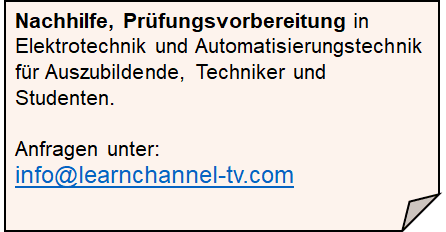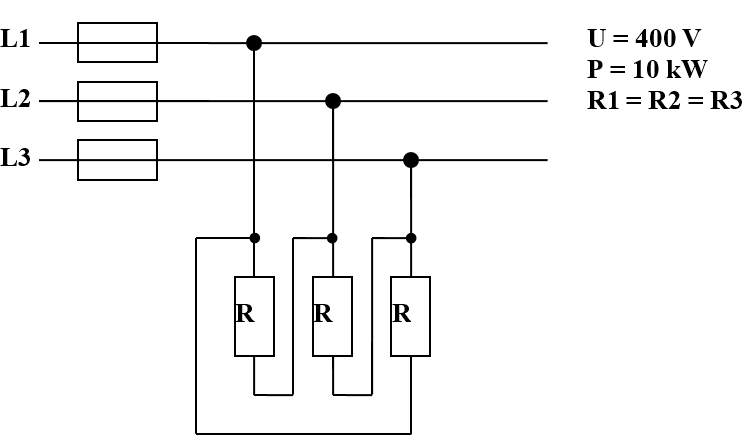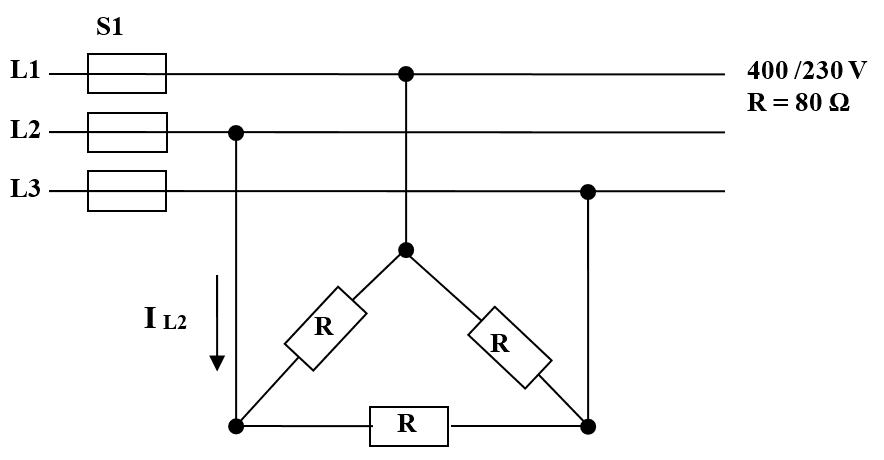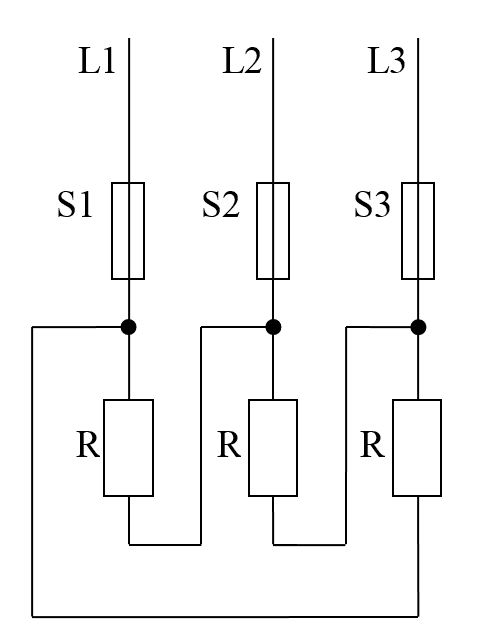(Deutsch)Learnchannel Nachhilfe

Part 1: Checkbox tasks - only one is right at a time!

What voltage can be measured on a 400 V TN-C-S system?

An industrial furnace is switched from Star to Delta. How does the power behave?

You have to connect a motor to a 400V 3-phase power supply. Which statement is not correct?

Part 2: Work orders

Work order 1:3 Phase Voltage - work order 1

Three resistors are connected to a three-phase power supply.

1.1 In which type of circuit are these resistors connected to each other?
1.2 What is the current IL in the supply line ?
1.3 What is the size of a phase resistor if the total power of the circuit is 10 kW?

Work order 2:

Three resistors are connected together to Delta.3 Phase Voltage - work order 2

2.1 What is the current flowing through a resistor?
2.2 How large is the conductor current IL?
2.3 Calculate the total power of the circuit!
2.4 The fuse S1 blows. How large is the conductor current IL2? Draw the equivalent circuit diagram!

Work order 3:3 Phase Voltage work order 3

Three equal-sized 80 Ω heating resistors of a three-phase heater are connected as shown. The string current is 5 A.

3.1 Which connection is shown here?
3.2 To which line voltage must these resistors be connected? Justify by calculation!
3.3 What is the supply current I1?
3.4 What is the rated power of the heater?
3.5 What is the current in the supply line if a fuse blows?

_______________________

=>  Get the solutions

The solutions are password-protected. Please send an e-mail to

info@learnchannel-tv.com    ... if you want to get access.